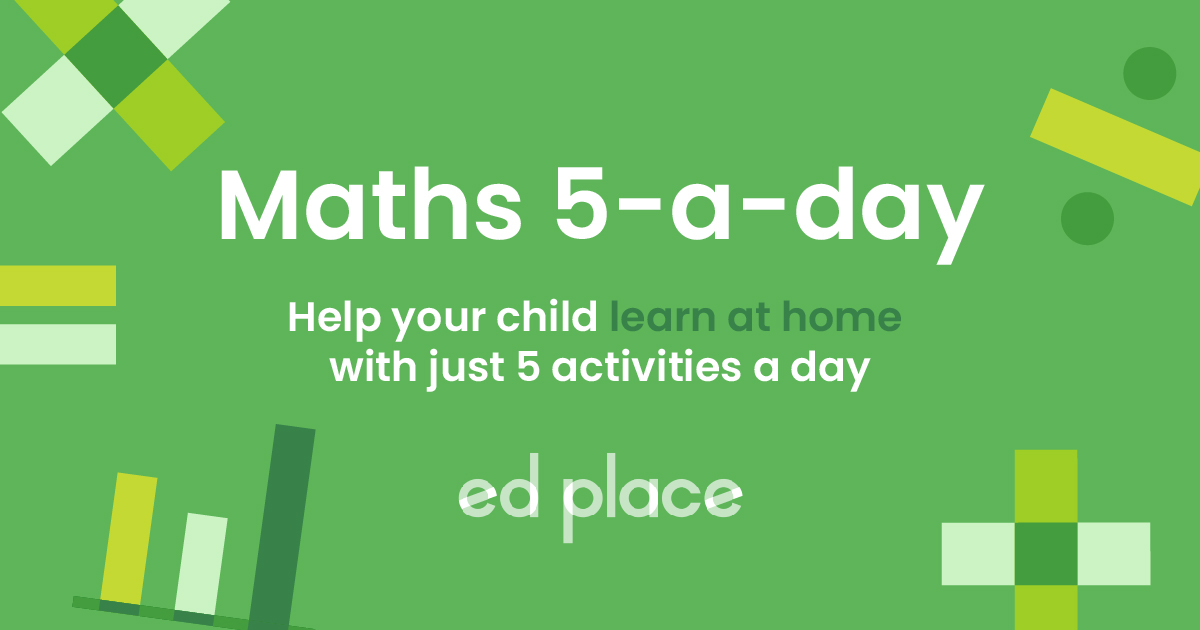# EdPlace's Year 6 Home Learning Maths Lesson: Multiplying & Dividing by Multiples of 10

Looking for short lessons to keep your child engaged and learning? Our experienced team of teachers have created English, maths and science lessons for the home, so your child can learn no matter where they are.  And, as all activities are self-marked, you really can encourage your child to be an independent learner. Get them started on the lesson below and then jump into our teacher-created activities to practice what they've learnt. We've recommended five to ensure they feel secure in their knowledge - 5-a-day helps keeps the learning loss at bay (or so we think!).

Are they keen to start practising straight away? Head to the bottom of the page to find the activities.

Now...onto the lesson!

Key Stage 2 Statutory Requirements for Maths
Year 6 students should be taught to multiply and divide numbers by 10, 100 and 1,000 giving answers up to 3 decimal places.

# Multiplying & Dividing by Multiples of 10

When multiplying any number (whole or decimal) by multiples of 10, there is an easy way to do it, which doesn’t involve using the ‘bus stop’ method! You just need to write the digits out and move them left or right across the place value columns.

Every single lesson taught in school has an objective that each child should achieve. We are confident that by the end of reading this you and your child will:

1) Understand how to multiply and divide numbers by 10, 100 and 1,000

2) Apply this understanding to independent work

3) Explain how they completed their work back to you!

## Step 1 - Understand key terminology

Place value – the value of each digit in a number.

Decimal point – the dot after the ones column (see Step 2 for an example) – it’s important to remember this because it shows that the digit(s) after the decimal point are only part of a whole number.

Decimal place – one of the columns after the decimal point – one decimal place is the tenths column, two decimal places is the hundredths column, three decimal places is the thousandths column (see Step 2 for an example).

A place holder is the number 0 to show there is nothing in that column.

## Step 2 - Check your child's prior understanding!

Your child will need to have a good understanding of place value and the names of the columns:## Step 3 - Introducing the new skill...

When we multiply, the number gets larger. Therefore, the digits move to the left.

When we multiply by 10, the digits move one space to the left (because there is one 0 in 10).37.5 &times; 10 = 375

When we multiply by 100, the digits move two spaces to the left (because there are two 0s in 100).29.05 &times; 100 = 2905

When we multiply by 1,000 the digits move three spaces to the left (because there are three 0s in 1000).54.87 &times; 1,000 = 54,870

We need to put a zero in the ones column as a place holder, to show that there are no ones.

The decimal point must always stay in the same place. It does not move. The digits move.

When we divide, the number gets smaller. Therefore, the digits move to the right.

When we divide by 10, the digits move one space to the right (because there is one 0 in 10).27.01 ÷ 10 = 2.701

When we divide by 100, the digits move two spaces to the right (because there are two 0s in 100).16.8 ÷ 100 = 0.168

Don’t forget to put a place holder in the ones column.

When we divide by 1,000 the digits move three spaces to the right (because there are three 0s in 1000).16 ÷ 1,000 = 0.016

This time, you need two place holders to show there are no ones or tenths.

## Step 4 - Putting it into practise...

Now have a go at these examples together:

a) 35.02 ÷ 10

b) 702 &times; 100

Challenge questions:

c) 8.48 &times; 1,000

d) 6.01 ÷ 100

## Step 5 - Activity time!

Now that you’ve covered how to multiply and divide by 10, 100 and 1,000 together, why not put this to the test and assign your child the following activities in this order? All activities are created by teachers and automatically marked. Plus, with an EdPlace subscription, we can automatically progress your child at a level that's right for them. Sending you progress reports along the way so you can track and measure progress, together - brilliant!